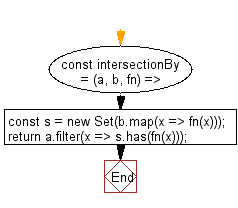# JavaScript: Get a list of elements that exist in both arrays, after applying the provided function to each array element of both

## JavaScript fundamental (ES6 Syntax): Exercise-210 with Solution

Write a JavaScript program to get a list of elements in both arrays, after applying the provided function to each array element of both.

• Use Array.prototype.filter() and Array.prototype.findIndex() in combination with the provided comparator to determine intersecting values

Sample Solution:

JavaScript Code:

``````//#Source https://bit.ly/2neWfJ2
const intersectionBy = (a, b, fn) => {
const s = new Set(b.map(x => fn(x)));
return a.filter(x => s.has(fn(x)));
};

console.log(intersectionBy([2.1, 1.2], [2.3, 3.4], Math.floor));
```
```

Sample Output:

```[2.1]
```

Flowchart:Live Demo:

See the Pen javascript-basic-exercise-210-1 by w3resource (@w3resource) on CodePen.

Improve this sample solution and post your code through Disqus

What is the difficulty level of this exercise?

Test your Programming skills with w3resource's quiz.

﻿